# Observer

The observer sees straight fence 100 m long in 30° view angle. From one end of the fence is 153 m.

How far is it from the another end of the fence?

Result

x =  179.9 m

#### Solution:Try calculation via our triangle calculator.

Leave us a comment of example and its solution (i.e. if it is still somewhat unclear...):Be the first to comment!#### To solve this example are needed these knowledge from mathematics:

Do you have a linear equation or system of equations and looking for its solution? Or do you have quadratic equation? Do you want to convert length units? See also our trigonometric triangle calculator.

## Next similar examples:Mast has 13 m long shadow on a slope rising from the mast foot in the direction of the shadow angle at angle 15°. Determine the height of the mast, if the sun above the horizon is at angle 33°. Use the law of sines.
2. Rotatable tower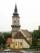Rotatable tower situated in the city center has ground shape of a regular polygon. If the tower is rotated by 14.4° around its centerpiece it looks from the side same. Your task is to calculate at least how many vertices can have a ground plan view of the.
3. AnglesIn the triangle ABC, the ratio of angles is: a:b = 4: 5. The angle c is 36°. How big are the angles a, b?
4. Angle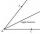Draw angle |∠ ABC| = 130° and built its axis. What angle is between axis angle and arm of angle?
5. Trapezium ABCDIn the figure, ABDC is a trapezium in which AB || CD. line segments RN and LM are drawn parallel to AB such that AJ=JK=KP. If AB=0.5m and AP=BQ=1.8m, find the lengths of AC, BD, RN and LM. angle D=angle C=60
6. Angles by cosine lawCalculate the size of the angles of the triangle ABC, if it is given by: a = 3 cm; b = 5 cm; c = 7 cm (use the sine and cosine theorem).
7. Scalene triangleSolve the triangle: A = 50°, b = 13, c = 6
8. Trapezium internal anglesA trapezium where AB is parallel to CD, has angle A : angle D = 4 :5, angle B = 3x-15 and angle C = 4x+20. Find angle A, B, C and D.
9. Theorem proveWe want to prove the sentence: If the natural number n is divisible by six, then n is divisible by three. From what assumption we started?
10. MedianThe median of the triangle LMN is away from vertex N 84 cm. Calculate the length of the median, which start at N.
11. What isWhat is the value of the smaller of a pair of numbers for which their sum is 78 and their division quotients are 0.3?
12. Two triangles SSA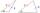Two triangles can be formed with the given information. Use the Law of Sines to solve the triangles. A = 59°, a = 13, b = 14
13. Gain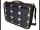Find the gain whenever the output power is 21x the input power.
14. Logarithmic equation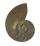Solve equation: log33(3x + 21) = 0
15. ReciprocalCalculate reciprocal of z=0.8-1.8i:
16. Ball gameRichard, Denis and Denise together scored 932 goals. Denis scored 4 goals over Denise but Denis scored 24 goals less than Richard. Determine the number of goals for each player.
17. Linear imaginary equationGiven that ? "this is z star" Find the value of the complex number z.